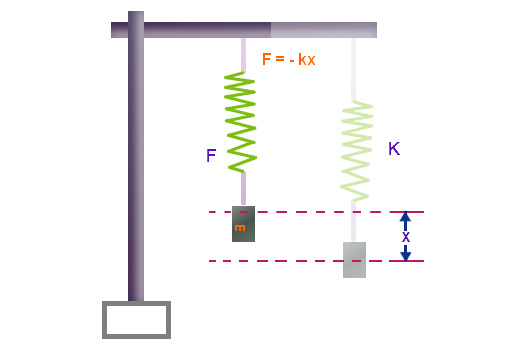# Spring Constant Formula

According to Hooke’s law, the force required to compress or extend a spring is directly proportional to the distance it is stretched.

## Formula of Spring Constant

The formula of spring constant is given as:

 Formula F=-k x SI unit N.m-1

Where,

• F is the restoring force of the spring directed towards the equilibrium
• k is the spring constant in N.m-1
• x is the displacement of the spring from its equilibrium position

In other words, the spring constant is the force applied if the displacement in the spring is unity. If a force F is considered that stretches the spring so that it displaces the equilibrium position by x.

## Spring Constant Dimensional Formula

We know that,

F=-kx

Therefore,

$$\begin{array}{l}k=-\frac{F}{x}\end{array}$$

Dimension of F=[MLT-2]

Dimension of x= [L]

Therefore, dimension of k=

$$\begin{array}{l}k=-\frac{[MLT^{-2}]}{[L]}=-[MT^{-2}]\end{array}$$The Spring Constant Formula is given as,

$$\begin{array}{l}k=-\frac{F}{x}\end{array}$$
where,

• F = Force applied,
• x = displacement by the spring
• The negative sign shows that the restoring force is opposite to the displacement

It is expressed in Newton per meter (N/m).

### Solved Examples

Example 1 A spring with load 5 Kg is stretched by 40 cm. Determine its spring constant.
Solution:
Given:
Mass m = 5 Kg

Displacement x = 40 cm

We know that,

Force F = m a = 5 × 0.4 = 2 N

The spring constant is given as:

$$\begin{array}{l}k=-\frac{F}{x}\end{array}$$

= – 2 / 0.4= – 5 N/m

Example 2 A boy weighing 20 pounds stretches a spring by 50 cm. Determine the spring constant of the spring.
Solution:
Given:
Mass m = 20 lbs = 20 / 2.2 = 9.09 Kg

Displacement x = 50 cm

The force F = ma = 9.09 × 9.8 = 89.082 N

The spring constant formula is given by:

$$\begin{array}{l}k=-\frac{F}{x}\end{array}$$

= – 89.082 / 0.5 = – 178.164 N/m.